# RFM Analysis Analysis Using Python

• Difficulty Level : Expert
• Last Updated : 08 Nov, 2021

In this article, we are going to see Recency, Frequency, Monetary value analysis using Python. But first, let us understand the RFM analysis briefly.

## What is RFM analysis?

RFM stands for recency, frequency, monetary value. In business analytics, we often use this concept to divide customers into different segments, like high-value customers, medium value customers or low-value customers, and similarly many others.

Let’s assume we are a company, our company name is geek, let’s perform the RFM analysis on our customers

1. Recency: How recently has the customer made a transaction with us
2. Frequency: How frequent is the customer in ordering/buying some product from us
3. Monetary: How much does the customer spend on purchasing products from us.

## Getting Started

Here we will import the required module( pandas, DateTime, and NumPy) and then read the data in the dataframe.

Dataset Used: rfm

## Python3

 `# importing necessary libraries` `import` `pandas as pd` `import` `datetime as dt` `import` `numpy as np`   `# importing the data` `df ``=` `pd.read_excel( < my excel ``file` `location > )` `df.head()`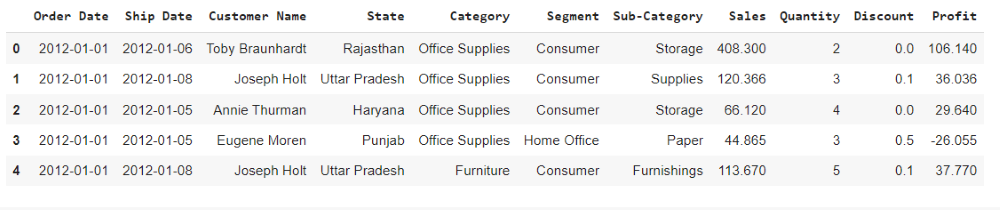### Calculating Recency

Here we are calculating recency for customers who had made a purchase with a company.

## Python3

 `df_recency ``=` `df.groupby(by``=``'Customer Name'``, ` `                        ``as_index``=``False``)[``'Order Date'``].``max``()` `df_recency.columns ``=` `[``'CustomerName'``, ``'LastPurchaseDate'``]` `recent_date ``=` `df_recency[``'LastPurchaseDate'``].``max``()` `df_recency[``'Recency'``] ``=` `df_recency[``'LastPurchaseDate'``].``apply``(` `    ``lambda` `x: (recent_date ``-` `x).days)` `df_recency.head()`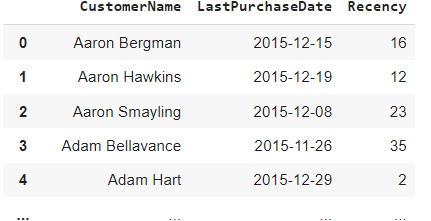### Calculating Frequency

We are here calculating the frequency of frequent transactions of the customer in ordering/buying some product from the company.

## Python3

 `frequency_df ``=` `df.drop_duplicates().groupby(` `    ``by``=``[``'Customer Name'``], as_index``=``False``)[``'Order Date'``].count()` `frequency_df.columns ``=` `[``'CustomerName'``, ``'Frequency'``]` `frequency_df.head()`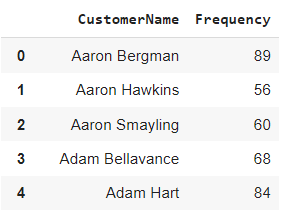### Calculating Monetary Value

Here we are calculating the monetary value of customer spend on purchasing products from the company.

## Python3

 `df[``'Total'``] ``=` `df[``'Sales'``]``*``df[``'Quantity'``]` `monetary_df ``=` `df.groupby(by``=``'Customer Name'``, as_index``=``False``)[``'Total'``].``sum``()` `monetary_df.columns ``=` `[``'CustomerName'``, ``'Monetary'``]` `monetary_df.head()`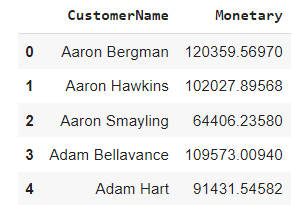### Merging all three columns in one dataframe

Here we are mering all the dataframe columns in a single entity using the merge function to display the recency, frequency, monetary value.

## Python3

 `rf_df ``=` `df_recency.merge(frequency_df, on``=``'CustomerName'``)` `rfm_df ``=` `rf_df.merge(monetary_df, on``=``'CustomerName'``).drop(` `    ``columns``=``'LastPurchaseDate'``)` `rfm_df.head()`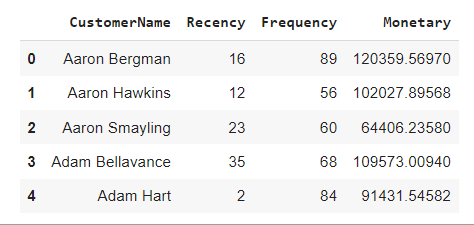### Ranking Customer’s based upon their recency, frequency, and monetary score

Here we are normalizing the rank of the customers within a company to analyze the ranking.

## Python3

 `rfm_df[``'R_rank'``] ``=` `rfm_df[``'Recency'``].rank(ascending``=``False``)` `rfm_df[``'F_rank'``] ``=` `rfm_df[``'Frequency'``].rank(ascending``=``True``)` `rfm_df[``'M_rank'``] ``=` `rfm_df[``'Monetary'``].rank(ascending``=``True``)`   `# normalizing the rank of the customers` `rfm_df[``'R_rank_norm'``] ``=` `(rfm_df[``'R_rank'``]``/``rfm_df[``'R_rank'``].``max``())``*``100` `rfm_df[``'F_rank_norm'``] ``=` `(rfm_df[``'F_rank'``]``/``rfm_df[``'F_rank'``].``max``())``*``100` `rfm_df[``'M_rank_norm'``] ``=` `(rfm_df[``'F_rank'``]``/``rfm_df[``'M_rank'``].``max``())``*``100`   `rfm_df.drop(columns``=``[``'R_rank'``, ``'F_rank'``, ``'M_rank'``], inplace``=``True``)`   `rfm_df.head()`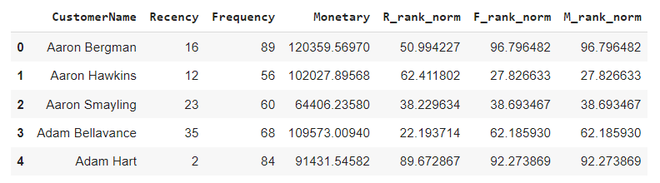### Calculating RFM score

RFM score is calculated based upon recency, frequency, monetary value normalize ranks. Based upon this score we divide our customers. Here we rate them on a scale of 5. Formula used for calculating rfm score is : 0.15*Recency score + 0.28*Frequency score + 0.57 *Monetary score

## Python3

 `rfm_df[``'RFM_Score'``] ``=` `0.15``*``rfm_df[``'R_rank_norm'``]``+``0.28` `*` `\` `    ``rfm_df[``'F_rank_norm'``]``+``0.57``*``rfm_df[``'M_rank_norm'``]` `rfm_df[``'RFM_Score'``] ``*``=` `0.05` `rfm_df ``=` `rfm_df.``round``(``2``)` `rfm_df[[``'CustomerName'``, ``'RFM_Score'``]].head(``7``)`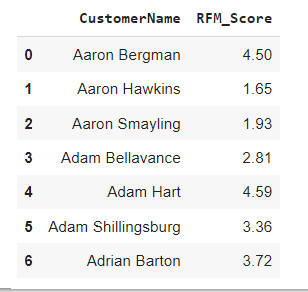### Rating Customer based upon the RFM score

• rfm score >4.5 : Top Customer
• 4.5 > rfm score > 4 : High Value Customer
• 4>rfm score >3 : Medium value customer
• 3>rfm score>1.6 : Low-value customer
• rfm score<1.6 :Lost Customer

## Python3

 `rfm_df[``"Customer_segment"``] ``=` `np.where(rfm_df[``'RFM_Score'``] >` `                                      ``4.5``, ``"Top Customers"``, ` `                                      ``(np.where(` `                                        ``rfm_df[``'RFM_Score'``] > ``4``,` `                                        ``"High value Customer"``,` `                                        ``(np.where(` `    ``rfm_df[``'RFM_Score'``] > ``3``,` `                             ``"Medium Value Customer"``,` `                             ``np.where(rfm_df[``'RFM_Score'``] > ``1.6``, ` `                            ``'Low Value Customers'``, ``'Lost Customers'``))))))` `rfm_df[[``'CustomerName'``, ``'RFM_Score'``, ``'Customer_segment'``]].head(``20``)`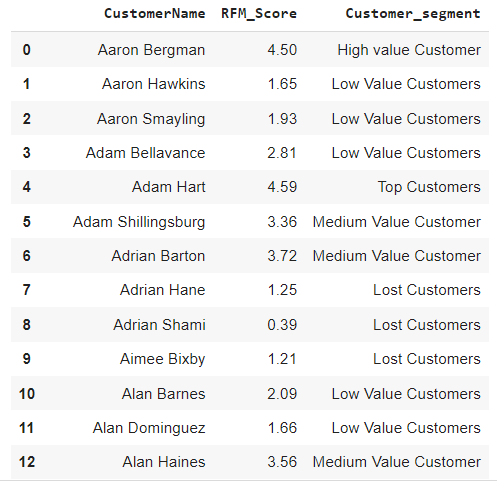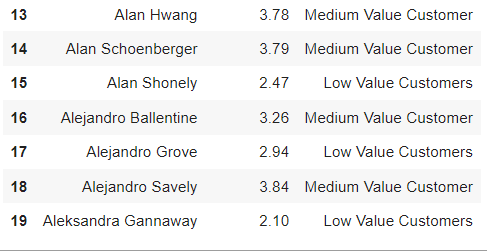### Visualizing the customer segments

Here we will use a pie plot to display all segments of customers.

## Python3

 `plt.pie(rfm_df.Customer_segment.value_counts(),` `        ``labels``=``rfm_df.Customer_segment.value_counts().index,` `        ``autopct``=``'%.0f%%'``)` `plt.show()`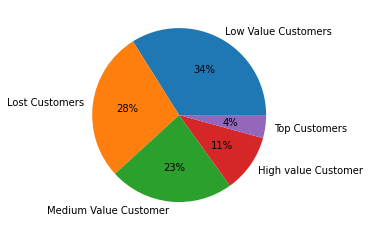My Personal Notes arrow_drop_up
Recommended Articles
Page :